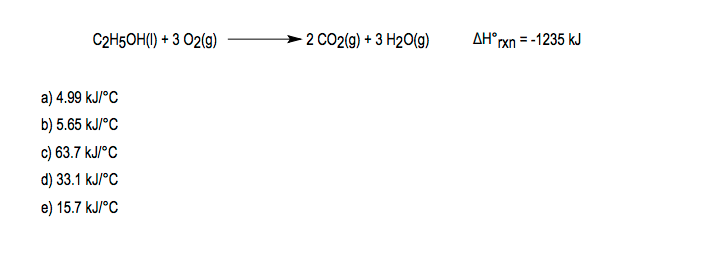# Problem: A 21.8 g sample of ethanol (C2H5OH) is burned in a bomb calorimeter, according to the following reaction. If the temperature rises from 25.0 to 62.3°C, determine the heat capacity of the calorimeter. The molar mass of ethanol is 46.07 g/mol.

###### FREE Expert Solution
92% (169 ratings)
###### Problem Details

A 21.8 g sample of ethanol (C2H5OH) is burned in a bomb calorimeter, according to the following reaction. If the temperature rises from 25.0 to 62.3°C, determine the heat capacity of the calorimeter. The molar mass of ethanol is 46.07 g/mol.What scientific concept do you need to know in order to solve this problem?

Our tutors have indicated that to solve this problem you will need to apply the Thermochemical Equation concept. You can view video lessons to learn Thermochemical Equation. Or if you need more Thermochemical Equation practice, you can also practice Thermochemical Equation practice problems.

What is the difficulty of this problem?

Our tutors rated the difficulty ofA 21.8 g sample of ethanol (C2H5OH) is burned in a bomb calo...as medium difficulty.

How long does this problem take to solve?

Our expert Chemistry tutor, Jules took 4 minutes and 4 seconds to solve this problem. You can follow their steps in the video explanation above.

What professor is this problem relevant for?

Based on our data, we think this problem is relevant for Professor St Hilaire's class at UM.